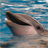# QlikView App Dev

Discussion Board for collaboration related to QlikView App Development.

Announcements
Read about the latest Qlik Community enhancements on the Community News blog!
cancel
Showing results for
Did you mean:Contributor II

## Help in Expression

Hello Everyone,

I have bar chart with 4 expressions and one Drill down Dimension(Country-State-Account). I have field called 'Status '.Expressions I gave

sum((if([Status]='Open',1,0))) - for Open Account

'Percentage Open='&Num((sum((if([Status]='Active',1,0)))/(Count([Account]))),'#,##0.0%')

=sum((if([Case Status]='Closed',1,0)))- For Closed Account

'Percentage Closed='&Num((sum((if(Status]='Closed',1,0)))/(Count([Account]))),'#,##0.0%')

Though I gave these 4 expressions in bar chart when I drill down to Account level - it is showing canceled Accounts too..

My requirement is - it should not involve canceled accounts. How to modify the formula to achieve this. Please help me asap. Thank you!

1 Solution

Accepted SolutionsCreator

Please have a look into set analysis.

example to count or sum facts based on a preselected deminsion.

=sum({<Status = {'Open'}>}<amount>)

where <amount>  is the field you want to sum. you of course also could use a count.Creator

Please have a look into set analysis.

example to count or sum facts based on a preselected deminsion.

=sum({<Status = {'Open'}>}<amount>)

where <amount>  is the field you want to sum. you of course also could use a count.Community Browser